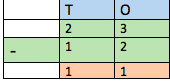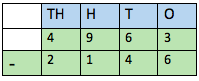# Subtraction: Lesson for Kids

Instructor: Sabrina Hairston

Sabrina has taught a variety of subjects and grades as a substitute teacher and will complete her MAT in 2016.

In this lesson, we'll discuss subtraction and the importance of this mathematical operation. You'll learn the steps of subtraction and learn how to borrow during subtraction. Then, test your knowledge with a short quiz!

## Why Is Subtraction Important?

Subtraction is an important tool we use to help us find out what is left when taking one number away from another. For example if Lauren has \$23 and spends \$12 on a new shirt, we can use subtraction to find out how much money Lauren has left. To set up this problem, we place the two numbers vertically, with the bigger number on top of the smaller number:It's important that the place values line up. The ones should be in the same column, the tens should be in the same column, and so on. Also, when writing subtraction problems, remember to add the subtraction sign to the left of the numbers. Once the numbers are lined up correctly, solve the problem by subtracting the bottom number from the top number in each column to get the answer of \$11. Lauren has \$11 left to spend.

## Working Through the Steps of Subtraction

Subtraction can be made easier by separating it into a few different steps. Let's work through these steps by solving another problem:

4,963 - 2,146 = ?

### Step 1: Set Up the Problem

To set up the subtraction problem vertically, put the larger number on top and the smaller number on bottom. In our problem, the larger number (4,963) comes first and the smaller number (2,146) goes under.

Always make sure the numbers line up based on their place value--the ones (O), tens (T), hundreds (H), and thousands (TH) all need to match up.

The problem looks like this:## Step 2: Borrow When Necessary

Sometimes, you can solve a problem from here by subtracting the bottom number from the top number along each column. But before we can solve this problem, we need to know about a third step that sometimes comes into play. Borrowing allows us to make a number bigger so that we can subtract from it.

To unlock this lesson you must be a Study.com Member.

### Register to view this lesson

Are you a student or a teacher?

#### See for yourself why 30 million people use Study.com

##### Become a Study.com member and start learning now.
Back
What teachers are saying about Study.com

### Earning College Credit

Did you know… We have over 200 college courses that prepare you to earn credit by exam that is accepted by over 1,500 colleges and universities. You can test out of the first two years of college and save thousands off your degree. Anyone can earn credit-by-exam regardless of age or education level.